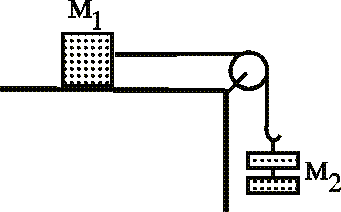Calculate (mu_s-mu_k)

Homework Statement

M1 has a mass of 6.510 kg. It is on a horizontal surface, connected by a light string to a hook. Mass M2 can be increased smoothly by adding masses little at a time.
The pulley has a negligible mass and no friction. When M2 is 3.281 kg it begins to accelerate downwards at a rate of 2.241 m/s2. Calculate (mu_s -mu_ k) for M1 on the surface.mu_s=(f_s)max/n

mu_k=f_k/n

The Attempt at a Solution

I really am unsure how to go about this problem. If someone could please guide me through it that would be greatly appreciated!

Draw a free body diagram for each box/crate to see where the forces are acting.

I did that. For M1 there is mg going down, a friction force to the left, normal force upwards, and tension to the right. For M2, there is mg going down, and tension going up.

Right, and you know that mu_s and mu_k are related by the equation F(friction)=mu*(F(normal))
and because this is a frictionless, massless pulley we are able to relate the tensions of the two boxes.

how do you calculate the normal force and friction force?

normal force for M1 should just equal 6.510kg x 2.241 m/s^2, right?

Help...anyone???

D H
Staff Emeritus
normal force for M1 should just equal 6.510kg x 2.241 m/s^2, right?
No. The mass M1 is not falling through the table, is it? What force is keeping that from happening?

See we cannot give you the answers.

Now you will have to consider two cases.

1) Where the block is on the verge on accelerating.

2) When it starts accelerating.

You will have to consider the frictional forces, tension in the string and the weights of the blocks and of course muk and mus.

I'm studying for my final and I'm having trouble solving this as well.....here is my work:

T_1 - u_s(m_1)g = m_1(a) = 0 (This equals zero because a = 0 when static)

T_2 = m_2(g)

T_1 = T_2

so

m_2(g) - u_s(m_1)g = 0

and static friction is:

f_s = u_s(m_?)(g)

I'm not given the m_1 when the object is at rest so how could I calculate both m_1 and u_s?

Ok....why is u_s= m_2/m_1